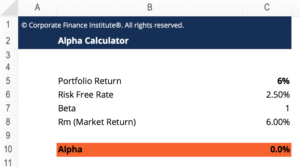# Alpha Calculator

## Alpha Calculator

This Alpha Calculator will compute the value of Alpha based on the Capital Asset Pricing Model. This CAPM-based approach will take the Portfolio Return, Risk Free Rate, Beta and Market Return and calculate Alpha from these elements:

Here is a snippet of the template Calculator:### Capital Assets Pricing Model (CAPM)

The CAPM formula is expressed as follows:

Therefore,

##### Alpha= R – Rf – beta (Rm-Rf)

Where:

R represents the portfolio return

Rf represents the risk-free rate of return

Beta represents the systemic risk of a portfolio

Rm represents the market return

### More Free Templates

For more resources, check out our business templates library to download numerous free Excel modeling, PowerPoint presentation, and Word document templates.

• Excel Modeling Templates
• PowerPoint Presentation Templates
• Transaction Document Templates

### Financial Analyst Certification

Become a certified Financial Modeling and Valuation Analyst (FMVA)® by completing CFI’s online financial modeling classes and training program!